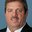Question-and-Answer Resource for the Building Energy Modeling Community
Get started with the Help page

# Rated sensible heat ratio, Sizing error

Hello, I'm trying to model an air to water heat pump with the object Coil:WaterHeating:AirToWaterHeatPump:Pumped. I did it a couple of times and I've always had problems to calculate rated sensible heat ratio.

This field is critical because I always get the error during simulation: "Calculated outlet air relative humidity greater than 1. The combination of rated air volume flow rate, total cooling capacity and sensible heat ratio yields coil exiting air conditions above the saturation curve. Possible fixes are to reduce the rated total cooling capacity, increase the rated air volume flow rate, or reduce the rated sensible heat ratio for this coil."

I saw this error here in other questions but I couldn't find a valid answer. It is defined as: "gross sensible cooling capacity divided by gross total cooling capacity".

My question is simply this: what cooling is it referring to? I'm trying to model a heat pump in heating mode. If E+ is considering (as I thought) the cooling of outside air (source side), to calculate it correctly I should have the absorbed heat from the outside evaporator but I've never seen this data in a technical sheet.

Is there a method to calculate it? Should I consider it as: Total cooling = Heating power CC - Electrical power PI ?

Considering Eurovent standard I have outdoor air with 85% RH so that with the total cooling (the only data I miss) it could be possible to calculate the SHR.

Thank you

edit retag close merge delete

Since I couldn't find the air flow, I set as Autosize. Then I tried every single value from 0.5 (the minimum) to 1 but I always got the same error. Then I tried to assume the outdoor air flow, I set it to 7000 m3/h (this is a small heat pump, 15.9 kW CC and 3.93 kW PI at 7°C). Then I calculated the ratio using 15.9-3.93=11.97 of Total cooling: I got a ratio of 0.7365 but it didn't work. Finally I calculated the ratio using 15.9 kW of Total cooling: I got a ratio of 0.691834 but it didn't work.

Does anyone have a different method?

I was successful

I calculated the ratio assuming the air flow to 7000 m3/h but I had to use: Total cooling = Heating power CC

I my case the ratio was calculated with Total cooling = 15.9 kW with a ratio of 0.7005578

Sort by » oldest newest most votedThe Coil:WaterHeating:AirToWaterHeatPump:Pumped represents a heat pump water heater so the likely information found in manufacturers literature would be for heating water. For this reason, the input of capacity for this object is total water heating capacity. The cooling coil used in the HPWH absorbs heat from ambient and is calculated as you describe, Total cooling = Heating power CC - Electrical power PI.

"Possible fixes are to reduce the rated total cooling capacity, increase the rated air volume flow rate, or reduce the rated sensible heat ratio for this coil."

For this coil, the easiest thing to do is lower the SHR without changing other inputs (although this might not represent the manufacturers product). To reduce the total cooling capacity you would lower the total heating rate or increase the power (lower the COP), or both.

more

Thank you very much, it's clear. Unfortunately I just realised I do not even have the air flow rate so that I cant' calculate the outlet air conditions (after the evaporator).

Just autosize all possible inputs and see how that works.

I assumed the air flow considering E+ limits of Ws/m3.

SHR worked with Total cooling = Heating power CC